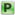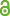Hauptmenü
• Autor
• Berkes, István
• Weber, Michel
• TitelOn series $$∑ c_k f (kx)$$ and Khinchin’s conjecture
• Datei
• Erschienen inIsrael Journal of Mathematics
• Band201
• Erscheinungsjahr2014
• Heft2
• Seiten593-609
• LicenceCC BY
• Zugriffsrechte• AbstractWe prove the optimality of a criterion of Koksma (1953) in Khinchin’s conjecture on strong uniform distribution. This verifies a claim of Bourgain (1988) and leads also to a near optimal a.e. convergence condition for series $$∑^{∞}_{k=1} c_k f (kx)$$ with $$f ∈ L^2$$ . Finally we show that under mild regularity conditions on the Fourier coefficients of $$f$$ , the Khinchin conjecture is valid assuming only $$f ∈ L^2$$ .